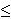## Hirsch Conjecture

The Hirsch conjecture states that the combinatorial diameter of every convex d-polytope with n facets is bounded by n-d.

Hirsch Conjecture (1957): Let P be a convex d-polytope with n facets. Then the diameter of the graph of the polytope P is at most n-d.

Many special cases of this conjecture have been verified, and in particular, the case of (0,1)-polytopes was settled by Naddef by an elegant proof in , and further generalized in . However, in the general case, the Hirsch conjecture is known only for d3. It is even open if there is a polynomial upper bound on the diameter. Kalai and Kleitman  found a quasi-polynomial bound by proving that the diameter is bounded by n2+log(d).

The special case of Hirsch Conjecture, the d-step conjecture, asserts that the diameter is equal to d if the polytope has precisely 2d facets. The d-step conjecture has been verified for d5, and similarly as the Hirsch Conjecture, it is completely open for higher dimensions. This has been the situation for the past 50 years, and there is no evidence for essential progress.

Bibliography:

 D. Naddef, The Hirsch conjecture is true for (0,1)-polytopes, Math. Progr. (Ser. B) 45 (1989) 109-110.

 T. Matsui and S. Tamura, Adjacency on combinatorial polyhedra, Discrete Appl. Math. 56 (1995) 311-321.

 G. Kalai and D. J. Kleitman, A quasi-polynomial bound for the diameter of graphs of polyhedra, Bull. Amer. Math. Soc. (N.S.) 26 (1992), 315-316.